# Gremlin recipes: 4 – Recursive traversals

This blog post is the 4th from the series Gremlin Recipes. It is recommended to read the previous blog posts first:

# I KillrVideo dataset

To illustrate this series of recipes, you need first to create the schema for KillrVideo and import the data. See here for more details.

The graph schema of this dataset is :

# II Graph expansion

In this post we will explore all the techniques for recursive traversal with Gremlin. We will focus on mainly on this part of the schema: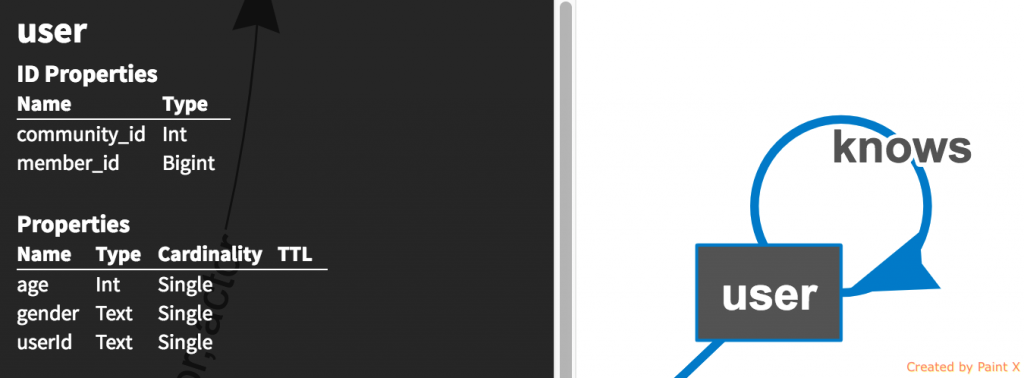Users-Knows

An user knows other users who knows other users etc. This is typical of a recursive traversal. First we want to know which user in our dataset knows the most other users:

```gremlin>g.V().                       // Iterator<Vertex>
hasLabel("user").                  // Iterator<User>
order().
by(outE("knows").count(), decr). // Order the users by their outgoing/incoming edge "knows", DESCENDING
values("userId").                  // Only display userId
limit(1)                           // Take the 1st matching user
==>u861

gremlin>g.V().                   // Iterator<Vertex>
has("user", "userId", "u861"). // Iterator<User>
outE("knows").                 // Iterator<Knows_Edge>
count()
==>13
```

So user u861 seems to knows 13 other users as his/her 1st degree connection.

Now let’s list all those 13 1st degree friends of user u861:

```gremlin>g.V().                   // Iterator<Vertex>
has("user", "userId", "u861"). // Iterator<User>
out("knows").                  // Iterator<User>
values("userId").              // Iterator<String>
fold()                         // Iterator<Collection<String>>
==>[u751, u868, u778, u887, u713, u733, u752, u548, u892, u657, u841, u150, u154]
```

# III Repeating traversal

Now, let’s display all 2nd degree friends of user u861:

```gremlin>g.V().                   // Iterator<Vertex>
has("user", "userId", "u861"). // Iterator<User>
as("u861").                    // Label u861
out("knows").                  // 1st degree friends
out("knows").                  // 2nd degree friends
dedup().                       // Remove duplicates
where(neq("u861")).            // Exclude u861
values("userId").              // Iterator<String>
fold()                         // Iterator<Collection<String>>
==>[u747, u719, u755, u756, u886, u880, u819, u831, u810, u832, u869, u718, u875, u830, u723, u727, u207, u744, u860, u704, u730, u829, u620, u882, u1035, u724, u800, u703, u705, u416, u717, u814, u767, u895, u804, u118, u263, u900, u887, u766, u851, u757, u606, u550, u155, u897, u750, u792, u797, u776, u702, u873, u855, u131, u196, u707, u103, u152]
```

As we can see, the combinatory explosion occurs right after the 2nd degree connection and will go on exponentially. We also notice the repetition of the step `out("knows")`. If we were looking at Nth degree connection, we would have to repeat the same step N times, which is quite verbose. To avoid this Gremlin exposes a convenient step: `repeat(... innner traversal)`. The above traversal can be rewritten as:

```gremlin>g.V().                   // Iterator<Vertex>
has("user", "userId", "u861"). // Iterator<User>
as("u861").                    // Label u861
repeat(out("knows")).times(2). // 2nd degree friends
dedup().                       // Remove duplicates
where(neq("u861")).            // Exclude u861
values("userId").              // Iterator<String>
fold()                         // Iterator<Collection<String>>
==>[u747, u719, u755, u756, u886, u880, u819, u831, u810, u832, u869, u718, u875, u830, u723, u727, u207, u744, u860, u704, u730, u829, u620, u882, u1035, u724, u800, u703, u705, u416, u717, u814, u767, u895, u804, u118, u263, u900, u887, u766, u851, u757, u606, u550, u155, u897, u750, u792, u797, u776, u702, u873, u855, u131, u196, u707, u103, u152]
```

So far so good. There are 58 users connected to u861 by 2nd degree connection.

# IV Emitting traversed vertices

Now, what if we want to display 1st and 2nd degree connections together ? For this Gremlin provides the `emit()` modulator to “output” all the traversed vertices:

```gremlin>g.V().                   // Iterator<Vertex>
has("user", "userId", "u861"). // Iterator<User>
as("u861").                    // Label u861
repeat(out("knows")).times(2). // 2nd degree friends
emit().                        // Output the traversed User vertices
dedup().                       // Remove duplicates
where(neq("u861"))
==>...
```

Using Datastax Studio for a better visualisation of the results, here is the web of all connections up to 2nd degree for user u861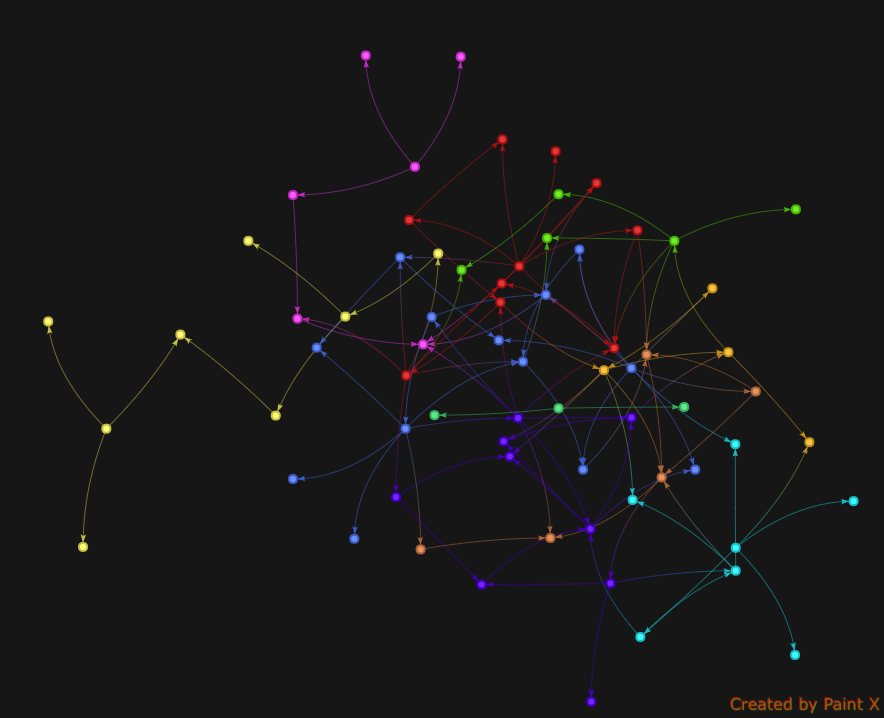2nd_degree_connections

The visualisation reveals the very fast graph expansion when using recursive traversals. We can restrict ourselves to the 1st degree connections:

```gremlin>g.V().                   // Iterator<Vertex>
has("user", "userId", "u861"). // Iterator<User>
repeat(out("knows")).times(1). // 1st degree friends
emit()
==>...
```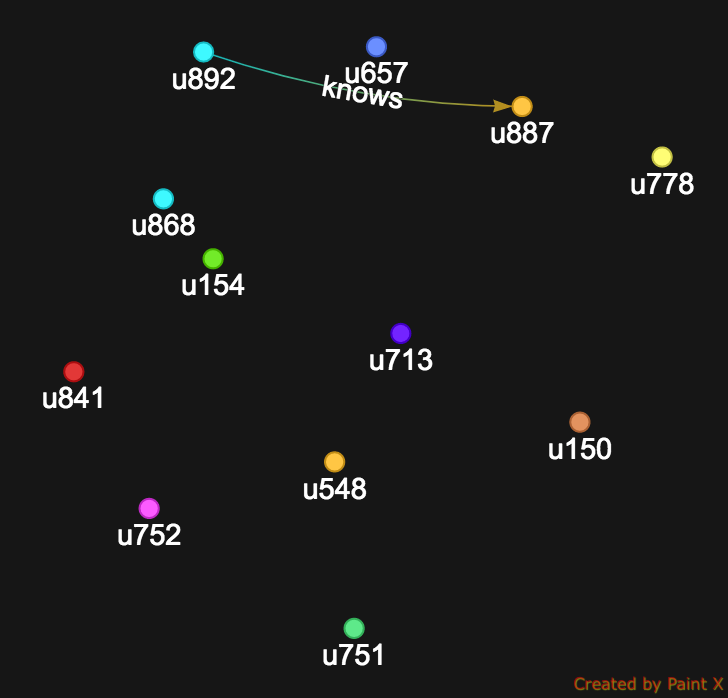1st_degree_connections

Not only the users are displayed but Datastax Studio conveniently shows all the edges that exist between the users and we just realize that u892 and u887 know each other !

But there is still a missing piece in our picture, where is u861 ??? Indeed, there are 2 possible placements for the `emit()` modulator. If `emit()` is placed after `repeat()`, it will “output” all vertices leaving the repeat-traversal. If `emit()` is placed before `repeat()`, it will “output” the vertices prior to entering the repeat-traversal.

In our example we just need to put `emit()` right before `repeat(out("knows")).times(1)`:

```gremlin>g.V().                   // Iterator<Vertex>
has("user", "userId", "u861"). // Iterator<User>
emit().                        // Output u861 and other users
repeat(out("knows")).times(1)  // 1st degree friends
==>...
```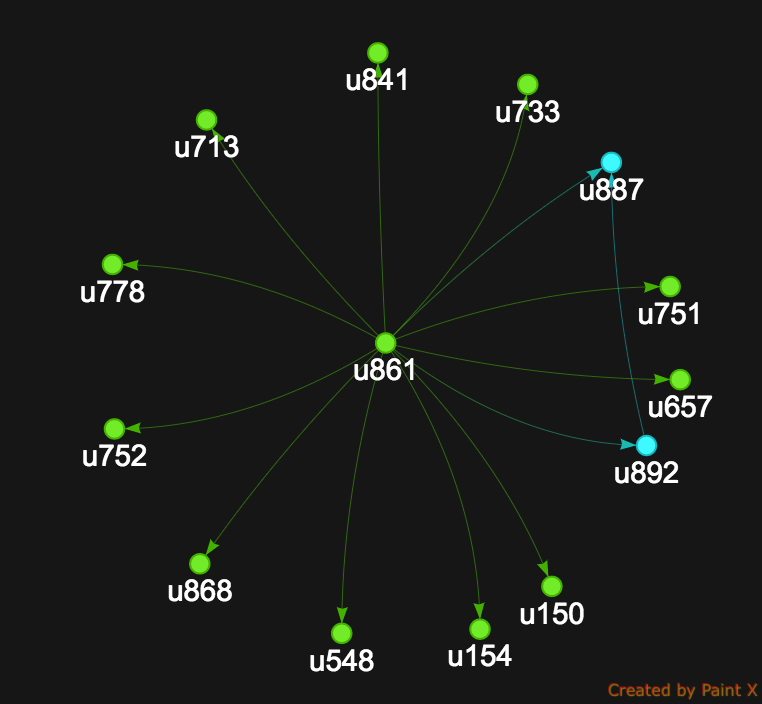Web_of_connections

Now, we have a beautiful web of connections outgoing from u861

# V Controlling the recursion

Until now, we were just using `times(x)` to control how far we will deep dive into recursion. What you should now is that `times(x)` is just a shorthand for a more generalized form of recursion control : `until(loops().is(eq(1)))`.

`until()` is controlling when to break out of the recursion based on a condition. As with `emit()` you can place `until()` before or after the `repeat()` step.

`until(...).repeat(...)` is equivalent to a `while(...) do{ } `repeat(….).until(…) is equivalent to `do{ } while(...)`

Suppose we want to know all friends of u861 having age 32:

```gremlin> :remote config timeout 10000
==>Set remote timeout to 10000ms
gremlin> g.V().
has("user", "userId", "u861").
until(has("age", 32)).
repeat(out("knows"))
Request timed out while processing - increase the timeout with the :remote command
Type ':help' or ':h' for help.
Display stack trace? [yN]
```

We just ran into a timeout. Why so ? Doesn’t use u861 have any connected friend with 32 years old ? We can run a quick check:

```gremlin> g.V().
has("user", "userId", "u861").
out("knows").
has("age", 32).
valueMap("userId", "age")
==>{userId=[u887], age=}
```

User u887 is indeed a direct connection of u861 having the matching age. So why did our traversal timeout ???

One problem with our traversal is that we may run into cyclic sub-graphs e.g user xxx which knows user yyy which knows in return user xxx. To shield ourselves from cyclic graph we can use `repeat(out("knows").simplePath()))` to consider only acyclic paths.

But the fundamental reason of the timeout is because Gremlin OLTP query engine is optimized for depth-first search. We will explain this concept is another post but long story shot, in the traversal `until(has("age", 32)).repeat(out("knows"))`, Gremlin will select one vertex and expand on the knows edge in multiple direction (graph explosion). Some branches may be stopped because the traverser encounters an user with age==32 but other branches may continue further to N hops. Using repeat(out(“knows”).simplePath())) helps reducing the combinatory explosion due to cyclic loops but we still have a rapid graph expansion.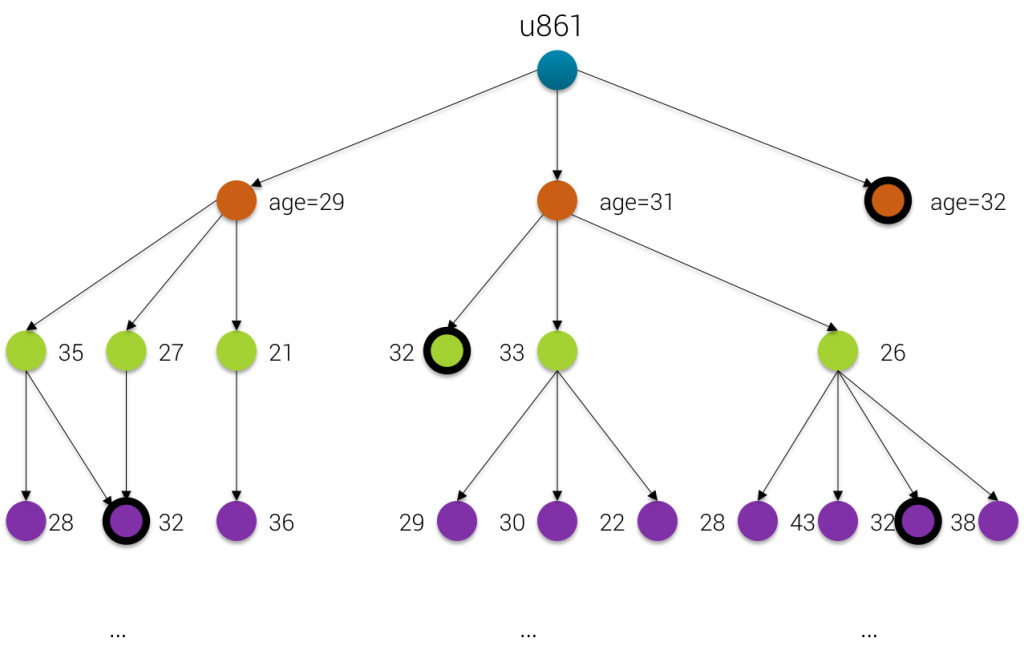Graph_Expansion

The only way to limit depth-first expansion is to control the depth of each traversed branch using the `loops()` step: `until(has("age", 32).or().loops().is(eq(3)))`

```gremlin>g.V().
has("user", "userId", "u861").
until(has("age", 32).or().loops().is(eq(3))).
repeat(out("knows").simplePath()).
valueMap("userId", "age")
==>{userId=[u887], age=}
==>{userId=[u719], age=}
==>{userId=[u755], age=}
==>{userId=[u800], age=}
==>{userId=[u887], age=}
==>{userId=[u361], age=}
==>{userId=[u740], age=}
...
```

Here we are ! The condition `until(has("age", 32).or().loops().is(eq(3)))` means that we will stop the loop whenever one of those conditions are met

• either an user with age == 32
• or the depth of the branch (materialized by `loops()`) has reached 3

That explains why in the results we can see some users with age == 32 (u887, u719 …) but other having age != 32 (u361, u740, …). They are still returned as a result because they match the 2nd condition of our `until()`

# VI Time-limiting the graph exploration

We have just seen that one technique to control the recursive combinatory explosion is to put a limit on the depth of each explored branch. Another powerful technique is to simply cap the computation time of graph expansion. For this we can use `timeLimit(time_in_millisecs)`. All the computation time, including the time taken by the loops and recursive traversal.

The previous traversal can be rewritten as:

```gremlin>g.V().
has("user", "userId", "u861").
until(has("age", 32)).
repeat(out("knows").simplePath()).
timeLimit(100).
valueMap("userId", "age")
==>{userId=[u887], age=}
==>{userId=[u719], age=}
==>{userId=[u755], age=}
==>{userId=[u800], age=}
==>{userId=[u887], age=}
```

In the above traversal, we want the computation of all the block `until(has("age", 32)).repeat(out("knows").simplePath())` will take at most 100ms. If we move the `timeLimit(100)` inside the `repeat(...)` step:

```gremlin>g.V().
has("user", "userId", "u861").
until(has("age", 32)).
repeat(out("knows").simplePath().timeLimit(100)).
valueMap("userId", "age").
dedup()
==>{userId=[u887], age=}
==>{userId=[u719], age=}
==>{userId=[u755], age=}
==>{userId=[u800], age=}
==>{userId=[u885], age=}
==>{userId=[u877], age=}
==>{userId=[u807], age=}
==>{userId=[u771], age=}
==>{userId=[u783], age=}
==>{userId=[u813], age=}
==>{userId=[u431], age=}
==>{userId=[u775], age=}
==>{userId=[u839], age=}
==>{userId=[u988], age=}
==>{userId=[u936], age=}
==>{userId=[u970], age=}
==>{userId=[u1088], age=}
==>{userId=[u1089], age=}
```

Now we have more result, in fact `repeat(out("knows").simplePath().timeLimit(100))` means that each of the repetition (loop) should take maximum 100ms

This time limiting step is quite powerful because when you don’t know how fast your graph will expand because each vertex may have a very different adjacency degree, using `timeLimit(...)` will keep your computation resources under control.

And that’s all folks! Do not miss the other Gremlin recipes in this series.

If you have any question about Gremlin, find me on the datastaxacademy.slack.com, channel dse-graph. My id is @doanduyhai

1.zhibo

Thanks for your post! The embedded Graph Schema Viewer is neat and useful. How did you create the killr_video_small.json file used by the schema viewer?

1.doanduyhai (Post author)

The killr_video_small.json file has been generated for me by the Datastax Studio team. So I guess it’s something open source people cannot get easily.

Anyway, there is nothing really complicated, it’s just some embedded Javascript to make the visualisation more user-friendly

1.zhibo

Thanks for your quick response! If feasible, could you kindly share the content of killr_video_small.json? That would help us to put the graph schema into the right format to utilize this schema viewer.

1.doanduyhai (Post author)

The link to killr_video_small.kryo is here: https://s3.amazonaws.com/datastax-graph-schema-viewer/index.html#/?schema=killr_video_small.json

It’s a kind of webapp that point to the JSON file located somewhere in Datastax S3 account. I can’t help more than that, I got it generated for me

1.zhibo

got it. thanks!

This site uses Akismet to reduce spam. Learn how your comment data is processed.